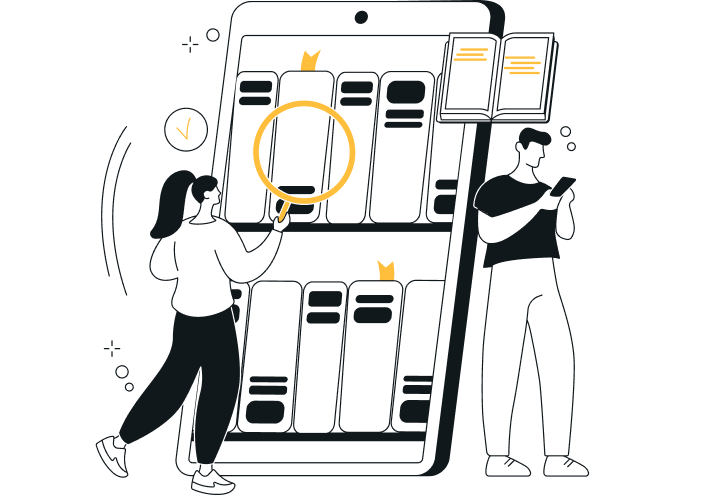# Abc Acquitsion

Category: Money
Last Updated: 19 Apr 2023
Pages: 3 Views: 221

The treasury bill rate at the time was 5. 8%, and the treasury bond rate was 6. 4%. The firm had debt outstanding of \$1. 7 billion and a market value of equity of \$1. 5 billion;  the corporate marginal tax rate was 36%.

• a. Estimate the expected return on the stock for a short term investor in the company.
• Order custom essay Abc Acquitsion with free plagiarism report450+ experts on 30 subjectsStarting from 3 hours delivery
Get Essay Help
• b. Estimate the expected return on the stock for a long? term investor in the company.
• c. Estimate the cost of equity for the company.

We use the CAPM:  The Expected Return on the stock = 0. 058 + 0. 95(0. 0792) = 0. 1332 = 13. 32%  Since the investor is a short? term investor, we use the bill rate, and the arithmetic mean. Since the focus is short? the term, we don’t need to take compounding into account.

Bond rate, and the geometric mean:  The expected return = 0. 064 + 0. 95(0. 0484) = 0. 1 or 11%, where 4. 84% is used as the estimate of the market risk premium since that is the geometric average of the market premium using the long? The cost of equity for the company is more appropriately the long?

Boise Cascade had debt outstanding of \$1. 7 billion and had a market value of equity of \$1. 5 billion; the corporate marginal tax rate was 36%.

• a. Assuming that the current beta of  0. 5  for the stock is a  reasonable one, estimate the unlevered beta for the company.
• b. How much of the risk in the company can be attributed to business risk and much to financial leverage?
• c. The levered beta of the company is given by the formula:

(levered)= (unlevered)(1+(1-tax rate)(D/E))

Solving, we get unlevered = 0. 95/(1+(1-0. 36)(1. 7/1. 5)) = 0. 55. The proportion of the risk of the firm’s equity that can be attributed to business risk is 0. 55/0. 95 = 58%, while the remainder is due to financial leverage risk. Genting  Berhad is a  Malaysian conglomerate,  with holdings in plantations and tourist resorts. The beta estimated for the firm, relative to the Malaysian stock exchange,  is  1. 15,  and the government borrowing rate in  Malaysia is 11. 5%. (Malaysian risk premium is 12%).

• a. Estimate the expected return on the stock.
• b. If you were an international investor,  what concerns if any,  would you have about using the beta estimated relative to the  Malaysian index? If you do, how would you modify the beta?
• c. The expected return on the stock, assuming that the marginal investor is a  Malaysian with primarily domestic holdings is 0. 115 + 1. 15(0. 12) = 25. 30%, using the risk premium based on country risk provided by rating agencies.
• d. For an international investor, who has the ability to diversify globally, some of the risks might be diversifiable, and hence the true beta might be lower. To take care of this possible overstatement, it would be appropriate to compute a beta relative to a more global index, such as the Morgan Stanley Capital Index.

You have just done a regression of monthly stock returns of Heavy Tech Inc.,  a manufacturer of heavy machinery, on monthly market returns over the last five years and come up with the following regression:

RHeavyTech=0. 5%+1. 2RM 2

The variance of the stock is 50%, and the variance of the market is 20%. The current T. bill rate is 3%. (It was 5% one year ago). The stock is currently selling for \$50, down \$4 over the last year, and has paid a dividend of \$2 during the last year and expects to pay a dividend of \$2. 50 over the next year. The New  York Stock Exchange (NYSE) composite has gone down 8% over the last year,  with a dividend yield of 3%. HeavyTech Inc. has a tax rate of 40%.

• a. What is the expected return on HeavyTech over the next year?
• b. What would you expect HeavyTech’s price to be one year from today?
• c. What would you have expected HeavyTech’s stock returns to be over the last year?
• d. What were the actual returns on HeavyTech over the last year?
• e. HeavyTech has \$100 million in equity and \$50 million in debt. It plans to issue  \$50  million in new equity and retire  \$50  million in debt. Estimate the new beta.